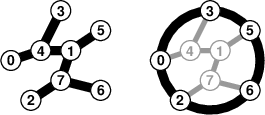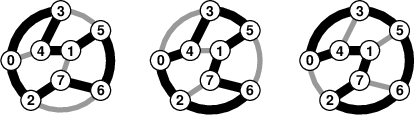Your browser (Unknown 0) is no longer supported. Some parts of the website may not work correctly. Please update your browser.

#### HamiltonianRoutesCount

Check whether a given sequence is a valid traversal of some ternary undirected tree.

An island has M towns: M ≥ 4 and M is an even integer. The towns are labelled with unique integers within the range [0..(M−1)]. The towns are connected through a network of (M−1) roads. Each road is bidirectional and connects exactly two distinct towns. Some towns, called cul-de-sacs, are connected to just one other town. Each of the remaining towns is connected to exactly three other towns. Each town can be reached from any other town.

Last year, the queen of the island went on a trip to visit all the towns (eventually arriving back at the starting point). She took a route that passed through each of (M−1) roads exactly twice. The sequence of towns visited during this trip was logged. Each cul-de-sac town was visited exactly once. Every other town had to be visited exactly three times (the final arrival at the starting point was not counted as a visit).

After last year's trip, the queen ordered the construction of a new circular highway connecting all cul-de-sac towns. The highway consists of a number of new roads, connecting the cul-de-sacs in the order of the queen's last visit.

The idea was raised that, with the new roads in place, it may be possible to devise a Hamiltonian route this year; i.e. one that passes through each town exactly once (and arrives back at the starting point).

Write a function

class Solution { public int solution(int[] A); }

that, given an array A consisting of N = 2*(M−1) integers representing the sequence in which M towns were visited last year, returns the number of different Hamiltonian routes possible this year.

The function should return 0 if no Hamiltonian route exists.
The function should return −1 if the number of possible Hamiltonian routes exceeds 100,000,000.
The function should return −2 if the route described by the array A violates any of the following conditions:

• each road connects distinct towns;
• each town is visited either exactly once or exactly thrice;
• each road is taken exactly twice.

Two Hamiltonian routes that contain exactly the same roads are considered the same, and are counted as one. For example, a route that goes through 0, 3, 4, 1, 5, 6, 7, 2 (and back to 0) is the same as the route 3, 4, 1, 5, 6, 7, 2, 0 (and back to 3), and is the same as the route 0, 2, 7, 6, 5, 1, 4, 3 (and back to 0).

For example, consider array A consisting of fourteen elements such that:

A = 4 A = 0 A = 4 A = 3 A = 4 A = 1 A = 5 A = 1 A = 7 A = 6 A = 7 A = 2 A = 7 A = 1

Given this array, the function should return 3, as explained below.

The left picture below shows the road network inferred from the sequence A, and the right picture shows the new circular highway that runs through the cul-de-sacs:Exactly three distinct Hamiltonian routes are possible:Taking another example, for the following array A:

A = 4 A = 4 A = 0 A = 3 A = 4 A = 1 A = 5 A = 1 A = 7 A = 6 A = 7 A = 2 A = 7 A = 1

the function should return −2, because the first road in the route does not connect distinct towns (it connects town 4 with itself).

Taking another example, for the following array A:

A = 3 A = 6 A = 3 A = 1 A = 5 A = 1 A = 0 A = 1 A = 3 A = 7 A = 3 A = 2 A = 3 A = 4

the function should return −2, because the given route violates the condition regarding towns (town 3 is visited neither exactly once, nor exactly thrice).

Taking another example, for the following sequence A:

A = 4 A = 0 A = 5 A = 0 A = 3 A = 0 A = 1 A = 3 A = 2 A = 3

the function should return −2, because the given route violates the condition regarding roads: the road connecting towns 0 and 3 occurs twice as it should (it is first taken in the direction from 0 to 3, then from 3 to 0), but the road connecting towns 0 and 1 (and few other roads) incorrectly occurs only once.

Write an efficient algorithm for the following assumptions:

• N = 2*(M−1), where M is an even integer within the range [4..200,000];
• each element of the array A is an integer within the range [0..(M−1)].